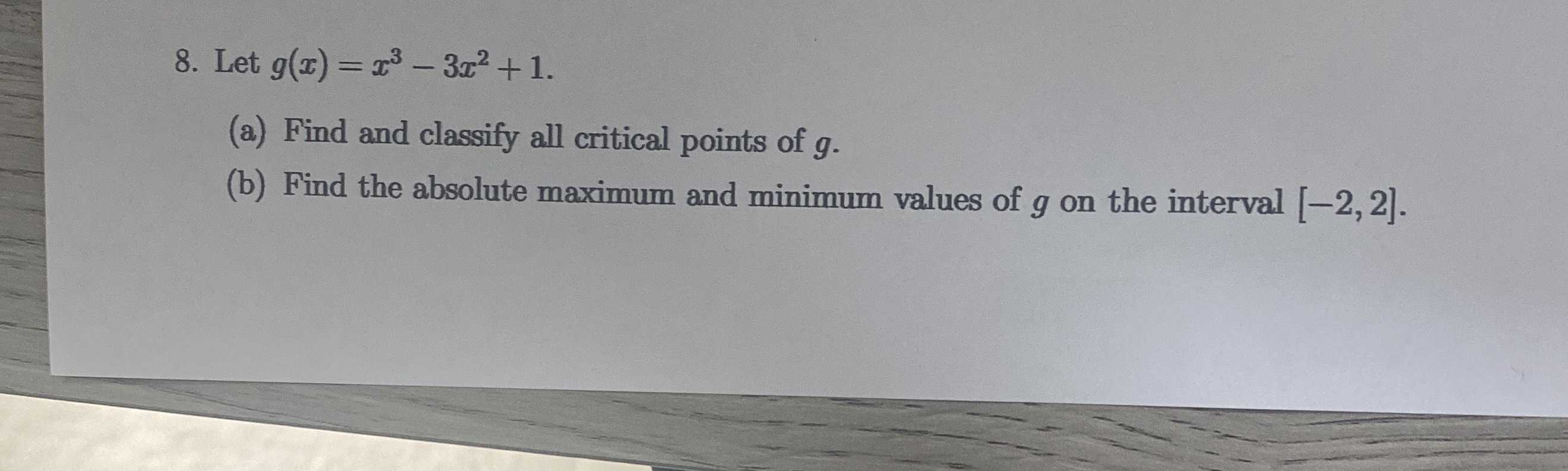### ¿Todavía tienes preguntas de matemáticas?

Pregunte a nuestros tutores expertos
Algebra
Pregunta8. Let $$g ( x ) = x ^ { 3 } - 3 x ^ { 2 } + 1 .$$

(a) Find and classify all critical points of $$g$$ .

(b) Find the absolute maximum and minimum values of $$g$$ on the interval $$[ - 2,2 ]$$ .